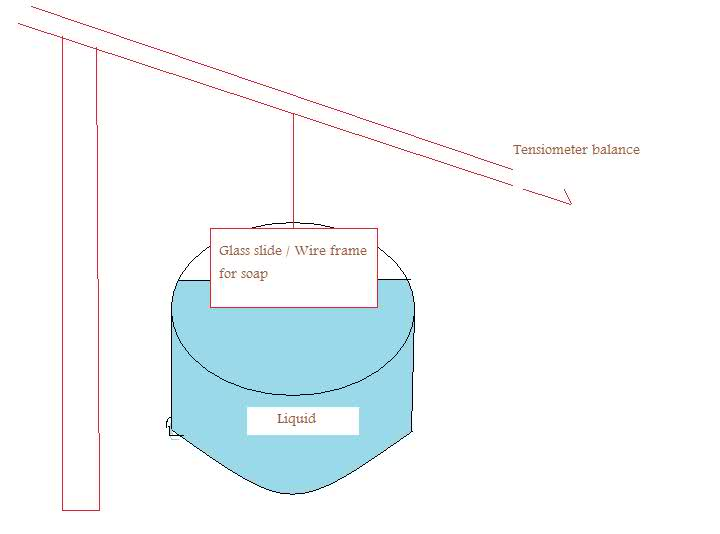# Some Surface Tension questions

Hello :)

So I am doing a lab to measure the surface tension of tap water, distilled water, and soap solution usin 3 different methods (well 2 for the waters and 3 for the soap).

I did this in a pretty standard way....attached a glass microscope slide to a tensiometer balance...made the slide stick to the surface of a liquid with only a thin film of liquid between them, and saw how far the pointer was depressed on the tensiometer. Then, I removed the liquid, and simply added weights to the slide until it was depressed to the same point - as to produce a force due to gravity equivalent to the force due to surface tension.
From noting how many weights i have attached, I could calculate surface tension usin the relationship Mg (of the weights) = 2 x l x T, where l is the length of the slide, and T the surface tension. the factor of 2 comes form the slide having 2 sides.

My first question - how does the thickness of the glass slide come into effect? It's supposed to have some kind of effect on my measurements....but I ccan't figure out what effect it would have, considering it's not used in the equation to calculate surface Tension based on this bethod...

2nd question - For the soap solution, i measured the surface tension in an additional way thats exactly the same as the above mentioned one, except instead of a glass slide i used a wire frame, which i submerged and it formed a thin film of soap which held it down. After measuring the equivalent force of gravity, it turned out to be about 25% less than the one measured with the glass slide (yet the frame and the glass slide have the same length L). What is the reason that the frame method of measuring surface tension of soap produces a lower value than using the glass slide??? My calculations are different enough for me to think its more than just experimental error...so why are the 2 methods so different?

## Answers and Replies

LowlyPion
Homework Helper
I don't know if I am visualizing it correctly, but won't there be a meniscus to the sides and clinging to the bottom? If the bottom is thick (you placed it edgewise?) then there may be some contribution from that mightn't there?

I'm not sure that I visualize your second set up at all.

Here is a crudely-drawn microsoft paint sketch of the setup(if it doesn't work - URL : http://tinypic.com/view.php?pic=30svhqq&s=4)

I basically adjust the balance of the tensiometer until the slide "sticks" to the surface of the liquid when u make them touch/ The length of the slide was about 100mm. The thickness of it is 1.5mm. So does the thickness matter because it provides 2 more lines on which the surface tension can act? So would I need to actually consider the relation Mg = 2 x length x Tension + 2 x width x tension instead of just Mg = 2 x length x tension as i mentioned above? or does this not work since the 2 sets of 2 lines are perpendicular to each other?...

As for the other quesiton about why i get the 2 different measurements for the soap - the set up looks as pictured above with a wire frame in the place of the Glass Slide. I dip the wireframe fully so that it forms a thin film , and it holds down the tensiometer. Why does using a wire frame which forms a film, versus just a glass slide, produce such different results for surface tension of the liquid soap?

Last edited:
LowlyPion
Homework Helper
Just to be clear is that edgewise to the surface or flat?

its edgewise to the surface with the side of the slide htiting the surface being a long thin rectangle with sides 100mm and 1.5mm

LowlyPion
Homework Helper
And you adjust until it breaks the surface tension?

I adjust the tensiometer until the slide is close enough to the surface of the liquid so that when i push it down and touch them together, the slide just barely sticks to the surface and doesn't come up. I then measure how low the pointer of the tensiometer balance is. Then, i remove the dish of liquid from below, and instead add weights to the glass slide. I add them until the glass slide and weights have depressed the tensiometer balance to the same point that it was when the liquid was holdin the slide down. At that point, the surface tension force must be equivalent to the force due to gravity of the weights added to the slide. so, since the glass slide has 2 long lines of 100mm touchin the surface, i use the relationship Mg = 2 x 100 mm x Tension -, and then solve for tension.

That looks pretty similar other than us using Tension = F / 2l instead of F/l

Edit : never mind that method is that similar....it measures the length as the amount the water crawls up a slide but I measure the length as the length of the longest side of the glass slide..

LowlyPion
Homework Helper
Actually I was trying to understand your method. So in that case then the width of the edge of the slide will affect the amount of water that must be pulled up won't it? If it was very wide (thick) the amount of water adhering to the bottom would begin to swamp what adhered to the sides. The higher the water climbs, the greater the weight of the force isn't it?

As to using soap, a surfactant, then you would expect less adherence and less force I'd think.